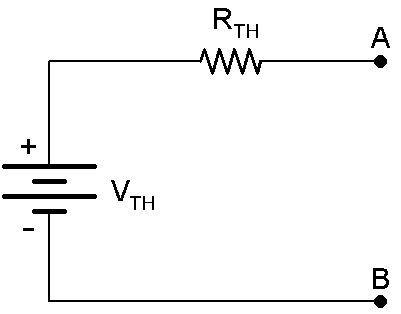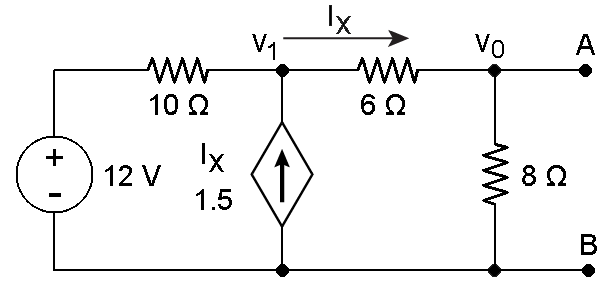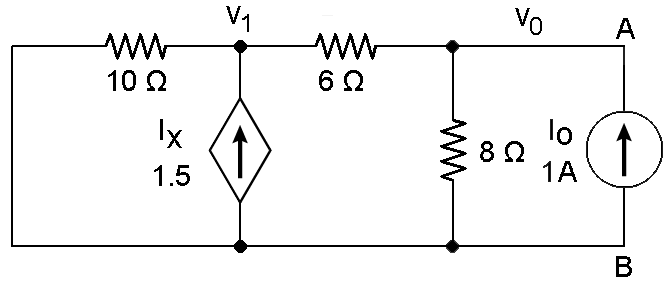Technical Article

# Learn About Thevenin Theorem and Dependent Source Circuits

October 02, 2015 by Kyle Marion

## Learn all about Thevenin's Theorem and its application in analyzing circuits with dependent sources.

Thevenin's Theorem and its application in analyzing circuits with dependent sources.

### Thevenin Theorem

When performing network analysis, Thevenin's theorem is a very helpful tool. It allows for a non-varying portion of a circuit to be replaced with a simplified design, thus simplifying the analysis of the entire network. This equivalent circuit performs the same way as the original circuit would.

Thevenin's theorem states that any linear, two-terminal portion of a network can be replaced by a Thevenin equivalent circuit. A Thevenin equivalent circuit consists of a voltage source (VTh) in series with a resistor (RTh) where VTh is the open-circuit voltage at terminals A-B and RTh   is the equivalent resistance at terminals A-B. This equivalent circuit can be seen in Figure 1 below. It is important to replace independent sources with their internal resistances when solving for RTh, i.e. current sources are replaced with open circuits and voltage sources are replaced with short circuits.Figure 1

#### Dependent Sources and Thevenin's Theorem

Thevenin's theorem can be applied when analyzing a circuit with dependent sources. In this case, all independent sources are turned off and the RTh is calculated by applying a current source or voltage source at the open terminal. When using a voltage source, it can be assumed to be 1V for simple calculations. Using mesh analysis, find the current Io at the output. When using a current source, it can be assumed to be 1A for easy calculations as well. Nodal analysis can be used to find the voltage at the terminal. The equivalent resistance then becomes a simple Ohm's law calculation, seen in Equation 1. If RTh takes a negative value, it means the circuit is supplying power to the terminals. Then to find VTh, use mesh analysis with all independent/dependent sources included and solve for the open circuit voltage.

$$R_{Th}=\frac{1\text{ } V}{I_{o}} \; \; \text{[Equation 1]}$$

Now to apply this theory to an example problem.Figure 2

Solving for RTh first, redraw the circuit with the 12V source as a short circuit. Then excite the circuit using either a 1A current source or 1V voltage source at the a-b terminal. Using a current source at the a-b terminal produces the circuit in Figure 3 below.Figure 3

Now to write the nodal analysis equations.

$$\frac{V_{1}}{10\text{ }\Omega}-1.5*I_{x}+I_{x}=0\; \; \text{[Equation 2]}$$

$$\frac{V_{o}-V_{1}}{6\text{ }\Omega}+\frac{V_{o}}{8\text{ }\Omega}=1\text{ }A\; \;\; \;\;\text{ [Equation 3]}$$

where,

$$I_{x}=\frac{V_{1}-V_{o}}{6\text{ }\Omega}\; \; \; \;\; \;\; \;\; \;\; \;\;\; \;\; \;\;\text{[Equation 4]}$$

Solving for Vyields V= .888V or 888.8mV. Remember that RTh = V/ Io, so RTh is equal to 888.8 mOhms.

Next, to find VTh, replace the 12V source and remove the current source from the a-b terminal. Again, utilizing nodal analysis, the equations are as follows.

$$\frac{V_{1}}{14\text{ }\Omega}+\frac{V_{1}-12}{10\text{ }\Omega}-1.5*\frac{V_{1}}{14\text{ }\Omega}=0\; \; \text{ [Equation 5]}$$

$$\frac{V_{Th}}{8\text{ }\Omega}-\frac{V_{1}-V_{Th}}{6\text{ }\Omega}=0\; \; \; \;\; \;\; \;\; \;\; \;\;\; \;\; \;\;\; \;\; \;\;\text{[Equation 6]}$$

Solving for Vin Equation 6 yields V= 1.75*VTh. Plugging this into Equation 5 and solving for VTh yields VTh = 10.66V.

This theorem allows for the simplification of resistor and source configurations into to a one source and one resistor equivalent circuit. It is commonly used with varying loads, that way the load current and power dissipation can be calculated easily.•satish kurakula March 07, 2017

hie why how did you took vi/14 2 times in the equation V1/14 Ω+(V1−12)/10 Ω−1.5∗V1/14 Ω=0 [Equation 5]

Like.
•Daulet March 08, 2017
hi. Remember that we have depentent source here Ix. The term −1.5∗V1/14 Ω is -1.5 * Ix. And from above we know that Ix = V1/14Ω. Hope it helps.
Like.
• A
afsal June 28, 2017

How can i simplify a T network with a current source given in a branch and load resistor at the other branch in between which a resistor and a dependent source is being connected in series to it?

Like.
•ABINANDHU M January 27, 2018

If the dependent source is (voltage control voltage source)or (CCVS) or (VCCS) ,what we have to do?

Like.
• Z
zorro_phu June 20, 2018

How come V0=0.888? When i slove it, it comes V0=-1.846…V.

Like.
• Z
zorro_phu June 20, 2018

How come V0=0.888? When i slove it, it comes V0=-1.846…V.

Like.
• Z
zorro_phu June 20, 2018
I used equation 2,3 and 4 to find V0.
Like.
•RK37 June 21, 2018
I am getting an answer similar to yours (-1.859 V). I'll ask another AAC technical editor to confirm, then we'll have to see if we can get this article sorted out.
Like.
•Mark Hughes June 21, 2018
If I ignore the article & diagrams, and focus strictly on the math, I can get to the 0.88888 answer. It'd be easier to demonstrate in Latex, but I don't think we can use that in comments. Substitute equation 4 into equation 2 yields V1=-5 V0. Substitute that result into equation 3 produces 0.88888, as the author stated. If, during the substitution, you lose track of your signs in the numerator of the first fraction of equation 3 (subtracting a positive number instead of subtracting a negative number), that produces the -1.846 value. I was unable to find the source of the -1.859 value, which leads me to believe it is the source of a rounding error. In my opinion, a larger issue exists with this article -- I do not believe this article clearly illustrates how to apply Thevenin's theorem. I'll discuss next steps with Robert via email.
Like.
•RK37 June 22, 2018
Thanks Mark. I looked at it again and I did indeed lose track of a minus sign.
Like.
• J
Janakaudara September 04, 2019

Can’t understand how find vth,anyone can explain

Like.